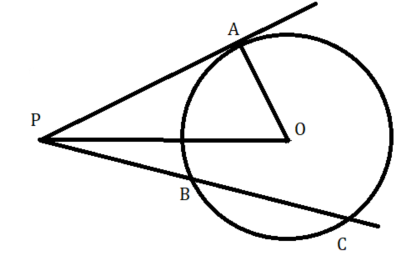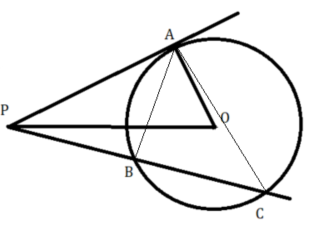GMAT Question of the Day: Daily via email | Daily via Instagram New to GMAT Club? Watch this Video

 It is currently 23 Jan 2020, 20:12### GMAT Club Daily Prep

#### Thank you for using the timer - this advanced tool can estimate your performance and suggest more practice questions. We have subscribed you to Daily Prep Questions via email.

Customized
for You

we will pick new questions that match your level based on your Timer History

Track

every week, we’ll send you an estimated GMAT score based on your performance

Practice
Pays

we will pick new questions that match your level based on your Timer History

#### Not interested in getting valuable practice questions and articles delivered to your email? No problem, unsubscribe here.# Question of the week - 28 (In the figure above, O is the center .....)

Author Message
TAGS:

### Hide Tags

e-GMAT RepresentativeV
Joined: 04 Jan 2015
Posts: 3219
Question of the week - 28 (In the figure above, O is the center .....)  [#permalink]

### Show Tags

1200:00

Difficulty:95% (hard)

Question Stats:25% (02:37) correct75% (03:24) wrongbased on 71 sessions

### HideShow timer Statistics

Question of the Week #28In the figure above, O is the center of the circle, with radius 10 units and PA is a tangent, whose length is equal to 24 units. If the lengths of PB and PC are distinct positive integers, and PB < PC, then find the sum of all possible lengths of PB?

A. 16
B. 18
C. 34
D. 58
E. 126

Attachment:QoW28.png [ 18.9 KiB | Viewed 1714 times ]

_________________

Originally posted by EgmatQuantExpert on 21 Dec 2018, 20:21.
Last edited by EgmatQuantExpert on 27 Feb 2019, 05:36, edited 3 times in total.
Math ExpertV
Joined: 02 Aug 2009
Posts: 8335
Re: Question of the week - 28 (In the figure above, O is the center .....)  [#permalink]

### Show Tags

1
3
EgmatQuantExpert wrote:In the figure above, O is the center of the circle, with radius 10 units and PA is a tangent, whose length is equal to 24 units. If the lengths of PB and PC are distinct positive integers, and PB < PC, then find the sum of all possible lengths of PB?

A. 16
B. 18
C. 34
D. 58
E. 126

It will be maximum when Pb~PC, that is PB is the tangent, and at that time it will be 24, so PB<24..
Now for Min, when PC lies on the hypotenuse itself...
So PO = √(24^2+10^2)=√676= 26, PB will be 26-10=16..
So min value is 16 and max is less than 24..

Now the property that will be useful here is (PA)^2=PB*PC...
So PB will be a factor of 24^2 but less than 24 and greater than or equal to 16..
So PB can be only 16 and 18..
Sum is 16+18=34

C
_________________
GMAT Club LegendV
Joined: 18 Aug 2017
Posts: 5723
Location: India
Concentration: Sustainability, Marketing
GPA: 4
WE: Marketing (Energy and Utilities)
Re: Question of the week - 28 (In the figure above, O is the center .....)  [#permalink]

### Show Tags

EgmatQuantExpert

EgmatQuantExpert wrote:In the figure above, O is the center of the circle, with radius 10 units and PA is a tangent, whose length is equal to 24 units. If the lengths of PB and PC are distinct positive integers, and PB < PC, then find the sum of all possible lengths of PB?

A. 16
B. 18
C. 34
D. 58
E. 126

Attachment:
QoW28.png
e-GMAT RepresentativeV
Joined: 04 Jan 2015
Posts: 3219
Re: Question of the week - 28 (In the figure above, O is the center .....)  [#permalink]

### Show Tags

Solution

Given:
• O is the center of the circle
• BC is a chord
• Radius of the circle = 10
• PA is the tangent, whose length = 24
• Lengths of PB and PC are integers, and PB < PC

To find:
• The sum of all possible lengths of PB

Approach and Working:
• Let us draw lines from A to B and from A to CNow, if we observe in triangles PAB and PAC,
• ∠APB = ∠APC (common angle)
• ∠PAB = ∠PCA (angles in alternate segments)

Therefore, we can say that the two triangles are similar,
• Thus, $$\frac{PA}{PB} = \frac{PC}{PA}$$
• $$PA^2 = PB * PC$$

Implies, PB * PC = $$24^2$$ (substituting the value of PA)
• Since, PB < PC, PB must be less than 24, and the minimum value that PB can take is when B is the intersection point of line PO and the circle.
• In such case, $$PB = PO – OB = √(PA^2 + OA^2) – 10 = √676 – 10 = 26 – 10 = 16$$
• Thus, 16 ≤ PB < 24, and PB must contain only 2 or 3 as its prime factors, since PB is a factor of $$24^2$$ and $$24^2 = 2^6 * 3^2$$

Therefore, the only possible values of PB that satisfies the above two conditions are PB = 16 or 18.

So, the sum of all possible lengths of PB = 16 + 18 = 34

Hence the correct answer is Option C.

_________________
Senior ManagerP
Joined: 03 Mar 2017
Posts: 369
Re: Question of the week - 28 (In the figure above, O is the center .....)  [#permalink]

### Show Tags

chetan2u wrote:
EgmatQuantExpert wrote:In the figure above, O is the center of the circle, with radius 10 units and PA is a tangent, whose length is equal to 24 units. If the lengths of PB and PC are distinct positive integers, and PB < PC, then find the sum of all possible lengths of PB?

A. 16
B. 18
C. 34
D. 58
E. 126

It will be maximum when Pb~PC, that is PB is the tangent, and at that time it will be 24, so PB<24..
Now for Min, when PC lies on the hypotenuse itself...
So PO = √(24^2+10^2)=√676= 26, PB will be 26-10=16..
So min value is 16 and max is less than 24..

Now the property that will be useful here is (PA)^2=PB*PC...
So PB will be a factor of 24^2 but less than 24 and greater than or equal to 16..
So PB can be only 16 and 18..
Sum is 16+18=34

C

That was a very comprehensive approach Chetan Sir._________________
--------------------------------------------------------------------------------------------------------------------------
All the Gods, All the Heavens, and All the Hells lie within you.
ManagerG
Joined: 12 Apr 2011
Posts: 149
Location: United Arab Emirates
Concentration: Strategy, Marketing
Schools: CBS '21, Yale '21, INSEAD
GMAT 1: 670 Q50 V31
GMAT 2: 720 Q50 V37WE: Marketing (Telecommunications)
Re: Question of the week - 28 (In the figure above, O is the center .....)  [#permalink]

### Show Tags

EgmatQuantExpert wrote:

Solution

Given:
• O is the center of the circle
• BC is a chord
• Radius of the circle = 10
• PA is the tangent, whose length = 24
• Lengths of PB and PC are integers, and PB < PC

To find:
• The sum of all possible lengths of PB

Approach and Working:
• Let us draw lines from A to B and from A to CNow, if we observe in triangles PAB and PAC,
• ∠APB = ∠APC (common angle)
• ∠PAB = ∠PCA (angles in alternate segments)

Therefore, we can say that the two triangles are similar,
• Thus, $$\frac{PA}{PB} = \frac{PC}{PA}$$
• $$PA^2 = PB * PC$$

Implies, PB * PC = $$24^2$$ (substituting the value of PA)
• Since, PB < PC, PB must be less than 24, and the minimum value that PB can take is when B is the intersection point of line PO and the circle.
• In such case, $$PB = PO – OB = √(PA^2 + OA^2) – 10 = √676 – 10 = 26 – 10 = 16$$
• Thus, 16 ≤ PB < 24, and PB must contain only 2 or 3 as its prime factors, since PB is a factor of $$24^2$$ and $$24^2 = 2^6 * 3^2$$

Therefore, the only possible values of PB that satisfies the above two conditions are PB = 16 or 18.

So, the sum of all possible lengths of PB = 16 + 18 = 34

Hence the correct answer is Option C.

I have a doubtIn the figure we know:

Triangle PAO = Right Angled Triangle
PA = 24
AO = 10
=> PO = 26

Now lets look at Triangle POC:

PO = 26
OC = 10
=> PC<PO + OC
=> PC < 26+10
=> PC < 36

Since PA*PA = PB*PC

For PC = 36
=> 24*24= 36*PB => PB = 16
Hence PB != 16

That leaves us with only 1 value i.e. PB = 18

Please let me know where I went wrong.

e-GMAT RepresentativeV
Joined: 04 Jan 2015
Posts: 3219
Re: Question of the week - 28 (In the figure above, O is the center .....)  [#permalink]

### Show Tags

1
eabhgoy wrote:

I have a doubtIn the figure we know:

Triangle PAO = Right Angled Triangle
PA = 24
AO = 10
=> PO = 26

Now lets look at Triangle POC:

PO = 26
OC = 10
=> PC<PO + OC
=> PC < 26+10
=> PC < 36

Since PA*PA = PB*PC

For PC = 36
=> 24*24= 36*PB => PB = 16
Hence PB != 16

That leaves us with only 1 value i.e. PB = 18

Please let me know where I went wrong.

Hi eabhgoy,

PC can be equal to OC + OP, when C is one of the end points of the diameter.

In that case PC = 36 and PB will be 36 - 20 = 16.

Regards,
_________________
ManagerG
Joined: 12 Apr 2011
Posts: 149
Location: United Arab Emirates
Concentration: Strategy, Marketing
Schools: CBS '21, Yale '21, INSEAD
GMAT 1: 670 Q50 V31
GMAT 2: 720 Q50 V37WE: Marketing (Telecommunications)
Re: Question of the week - 28 (In the figure above, O is the center .....)  [#permalink]

### Show Tags

EgmatQuantExpert wrote:
eabhgoy wrote:

I have a doubtIn the figure we know:

Triangle PAO = Right Angled Triangle
PA = 24
AO = 10
=> PO = 26

Now lets look at Triangle POC:

PO = 26
OC = 10
=> PC<PO + OC
=> PC < 26+10
=> PC < 36

Since PA*PA = PB*PC

For PC = 36
=> 24*24= 36*PB => PB = 16
Hence PB != 16

That leaves us with only 1 value i.e. PB = 18

Please let me know where I went wrong.

Hi eabhgoy,

PC can be equal to OC + OP, when C is one of the end points of the diameter.

In that case PC = 36 and PB will be 36 - 20 = 16.

Regards,

Thank you

So since OC is radius then it is always a part of a diameter chord.Re: Question of the week - 28 (In the figure above, O is the center .....)   [#permalink] 06 Jan 2019, 00:23
Display posts from previous: Sort by

# Question of the week - 28 (In the figure above, O is the center .....)